# Math in Focus Grade 2 Cumulative Review for Chapters 15 to 17 Answer Key

This handy Math in Focus Grade 2 Workbook Answer Key Cumulative Review for Chapters 15 to 17 detailed solutions for the textbook questions.

## Math in Focus Grade 2 Cumulative Review for Chapters 15 to 17 Answer Key

Concepts and Skills

Skip-count.

Question 1.
3, 6, 9, ________, ________, ________, ________, ________, ________, ________
3, 6, 9, 12, 15, 18, 21, 24, 27, 30.

Explanation:
I wrote the next seven numbers
I skip counted by 3 and wrote the numbers.
The numbers have difference of 3 between each consecutive pair.

Question 2.
4, 8, 12, ________, ________, ________, ________, ________, ________, ________
4, 8, 12, 16, 20, 24, 28, 32, 36, 40.

Explanation:
I wrote the next seven numbers
I skip counted by 4 and wrote the numbers.
The numbers have difference of 4 between each consecutive pair.

Fill in the blanks.

Question 3.
4 groups of 3 = ________ × _______ = _______
4 x 3 = 12

Explanation:
The product of 4 and 3 is 12
So, 4 x 3 that is 4 groups of 3 is 12.

Question 4.
8 groups of 3 = ________ × _______ = _______
8 x 3 = 24

Explanation:
The product of 8 and 3 is 24
So, 8 x 3 that is 8 groups of 3 is 24.

Question 5.
3 groups of 4 = ________ × _______ = _______
3 x 4 = 12

Explanation:
The product of 3 and 4 is 12
So, 3 x 4 that is 3 groups of 4 is 12.

Question 6.
9 groups of 4 = ________ × _______ = _______
9 x 4 = 36

Explanation:
The product of 9 and 4 is 36
So, 9 x 4 that is 9 groups of 4 is 36.

Use dot paper to find the missing numbers.

Question 7.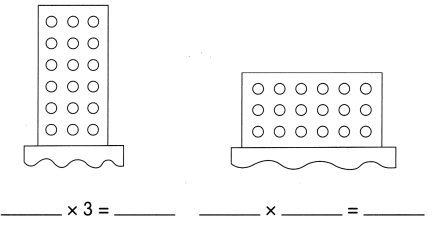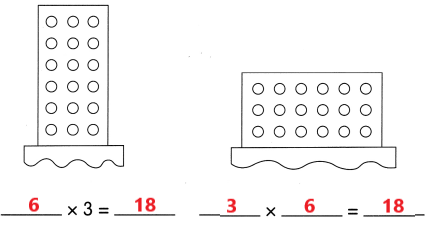Explanation:
I wrote the missing numbers in the equations using dot papers
There are 6 rows and 3 columns that is 6 groups of 3 dots
So, 6 x 3 = 18
In the second dot paper
There are 3 rows and 6 columns that is 3 groups of 6 dots
3 x 6 = 18.

Question 8.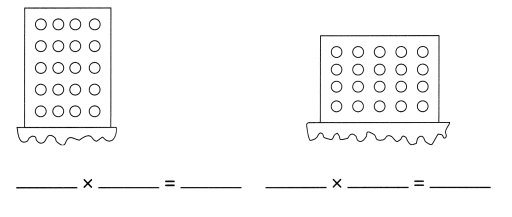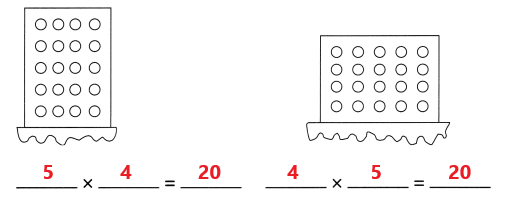Explanation:
I wrote the missing numbers in the equations using dot papers
There are 5 rows and 4 columns that is 5 groups of 4 dots
So, 5 x 4 = 20
In the second dot paper
There are 4 rows and 5 columns that is 4 groups of 5 dots
4 x 5 = 20.

Question 9.
9 × 3 = __________
3 × 9 = __________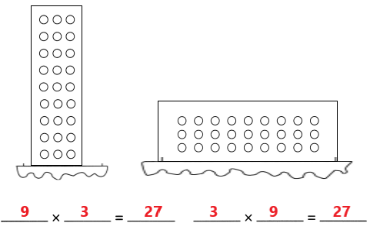Explanation:
I completed the multiplication sentences using dot papers
So, 9 x 3 = 27 and 3 x 9 = 27.

Question 10.
7 × 3 = __________
3 × 7 = __________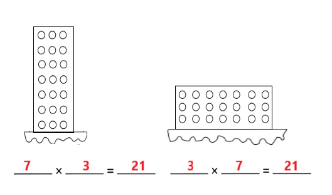Explanation:
I completed the multiplication sentences using dot papers
So, 7 x 3 = 21 and 3 x 7 = 21.

Question 11.
10 × 4 = _________
4 × 10 = _________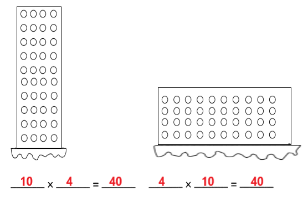Explanation:
I completed the multiplication sentences using dot papers
So, 10 x 4 = 40 and 4 x 10 = 40.

Question 12.
6 × 4 = __________
4 × 6 = __________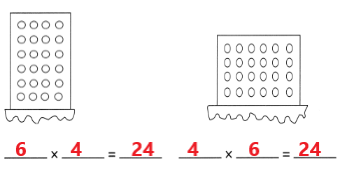Explanation:
I completed the multiplication sentences using dot papers
So, 6 x 4 = 24 and 4 x 6 = 24.

Fill in the blanks.

Question 13.
10 × 4 = __________
7 × 4 = 10 groups of 4 – 3 groups of 4
= _________ – 12
= _________
10 x 4 = 40
7 x 4 = 28

Explanation:
10 × 4 = __________
7 × 4 = 10 groups of 4 – 3 groups of 4
= 40 – 12
= 28
So, 7 x 4 = 28.

Question 14.
5 × 3 = __________ 7 × 3 = 5 groups of 3 + 2 groups of 3
= ________ + 6
= _________
5 x 3 = 15
7 x 3 = 21

Explanation:
7 × 3
= 5 groups of 3 + 2 groups of 3
= 15  + 6
= 21
So, 7 x 3 = 21.

Complete the multiplication and division sentences.

Question 15.
________ × 3 = 15
15 ÷ 3 = __________
5 x 3 = 15
15 ÷ 3 = 5

Explanation:
5 groups of 3 is 15
If 15 is divided into 3 groups then each group will have 5
So, 15 ÷ 3 = 5.

Question 16.
________ × 4 = 20
20 ÷ 4 = __________
5 x 4 = 20
20 ÷ 4 = 5

Explanation:
5 groups of 4 is 20
If 20 is divided into 4 groups then each group will have 5
So, 20 ÷ 4 = 5.

A large bottle contains different kinds of nuts. The graph shows the number of each kind of nut in the Jar.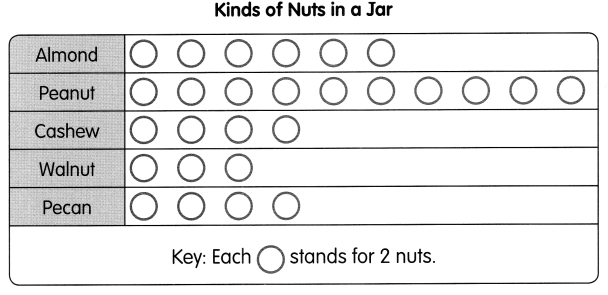Question 17.
There are __________ peanuts in the jar.
20 peanuts

Explanation:
There are 10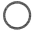for peanuts
Eachstands for 2 nuts
10 x 2 = 20
So, there are 20 peanuts in the jar.

Question 18.
The number of __________ and _________ in the jar is the same.
Cashew and Pecan

Explanation:
Eachstands for 2 nuts
There are 4for cashew and 4for pecans
4 x 2 = 8
So, The number of Cashew and Pecans in the jar is the same that is 8.

Question 19.
There are __________ fewer walnuts than almonds.
6 fewer walnuts

Explanation:
Eachstands for 2 nuts
There are 6for Almonds
There are 3for Walnuts
6 x 2 = 12
3 x 2 = 6
12 – 6 = 6
So, there are 6 fewer walnuts than almonds in the jar.

Question 20
There are __________ more peanuts than cashews.
12 more peanuts

Explanation:
Eachstands for 2 nuts
There are 4for cashew and 10for peanuts
4 x 2 = 8
10 x 2 = 20
20 – 8 = 12
So, there are 12 more peanuts than cashews in the jar.

Question 21.
There are ____________ almonds and pecans in all.
20 almonds and pecans

Explanation:
Eachstands for 2 nuts
There are 6for almonds and 4for pecans
6 x 2 = 12
4 x 2 = 8
12 + 8 = 20
So, there are 20 almonds and pecans in all in the jar.

Count the vegetables in a bin. Then complete the tally chart.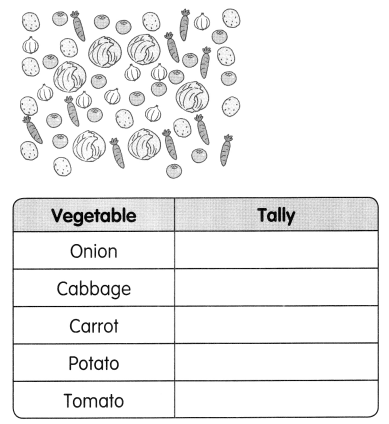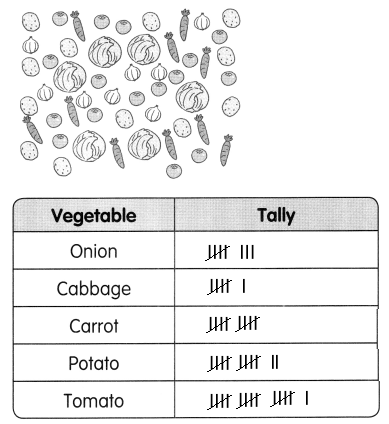Explanation:
I counted the number of each kind of vegetables and drew tally marks for the numbers.

Question 22.
Use the picture and the tally chart to make a bar graph.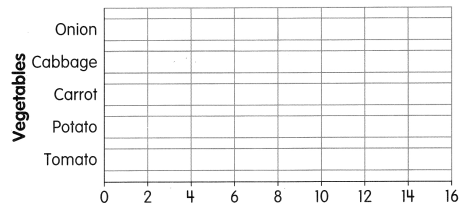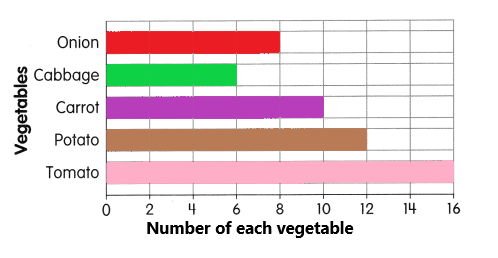Explanation:
I used the tally marks to fill the bar graph
I counted and filled the number of each kind of vegetable in the graph.

Question 23.
How many carrots are there?
10

Explanation:
There are 10 carrots.

Question 24.
How many tomatoes are there?
16

Explanation:
There are 16 tomatoes.

Question 25.
How many cabbages are there?
6

Explanation:
There are 6 cabbages.

Problem Solving

Question 26.
Alicia has 3 bags. She puts 10 beads in each bag. How many beads does she have?
There are _________ beads in all.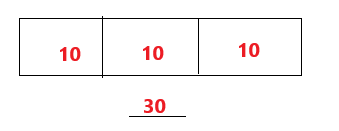Explanation:
Alicia has 3 bags
She puts 10 beads in each bag
10 x 3 = 30 or
10 + 10 + 10 = 30
There are 30 beads in all.

Question 27.
Peter has 18 crayons. He divides them into 3 equal groups. How many crayons are in each group?
There are ___________ crayons in each group.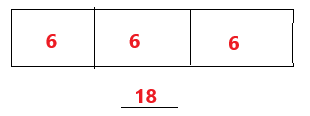Explanation:
Peter has 18 crayons
He divides them into 3 equal groups
18 ÷ 3 = 6
So, there are 6 crayons in each group.

Question 28.
Yasmin buys 30 stickers. She distributes the stickers equally among her 5 friends. How many stickers does each of her friends get?
Each friend gets _____________stickers.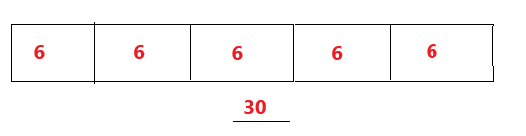Explanation:
She distributes the stickers equally among her 5 friends
6 + 6 + 6 + 6 + 6 = 30
Each friend gets 6 stickers.

Question 29.
A day camp has 12 soccer balls. Each team uses 3 balls. How many teams are there?
There are __________ teams.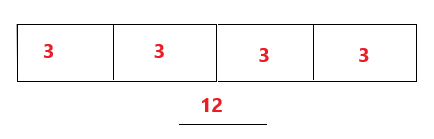Explanation:
A day camp has 12 soccer balls
Each team uses 3 balls
12 ÷ 3 = 4
There are 4 teams.

Question 30.
5 bags of food for a food drive have a mass of 25 kilograms. Each bag has the same mass. What is the mass of each bag?
The mass of each bag is ___________ kilograms.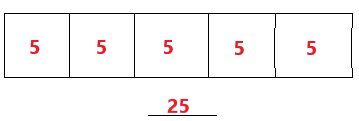Explanation:
5 bags of food for a food drive have a mass of 25 kilograms
25 ÷ 5 = 5
The mass of each bag is 5 kilograms.

Question 31.
The total length of a piece of lace is 28 feet. It is cut into equal pieces 4 feet long. How many pieces are there?
There are __________ pieces.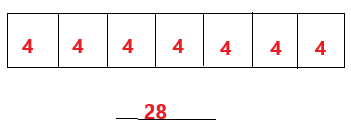Explanation:
The total length of a piece of lace is 28 feet
It is cut into equal pieces 4 feet long
24 ÷ 4 = 7 or
4 + 4 + 4 + 4 + 4 + 4 + 4 = 28 7 groups
There are 7 pieces.

Question 32.
Andrew uses 6 liters of water to water his plants every day. How many liters of water does he use in 4 days?
He uses ________ liters of water.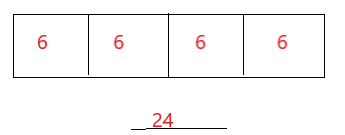Explanation:
Andrew uses 6 liters of water to water his plants every day
water for  4 days is 6 x 4 = 24 or
6 + 6+ 6 + 6 = 24
He uses 24 liters of water.

Complete the picture graph using Exercises 33 and 34. Then use the graph to solve Exercises 35 and 36.

A group of people attended a workshop. They were divided into 5 groups. The number of people in each group is shown in the graph.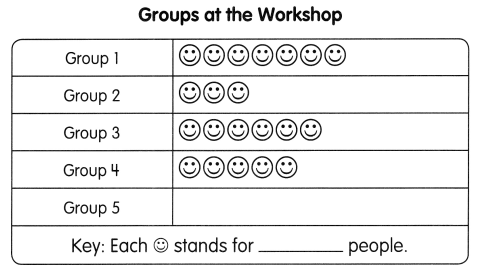Question 33.
There were 15 people in Group 2. What does each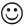stand for?
Eachstand for 5 people.

Question 34.
Drawto show 20 people in Group 5.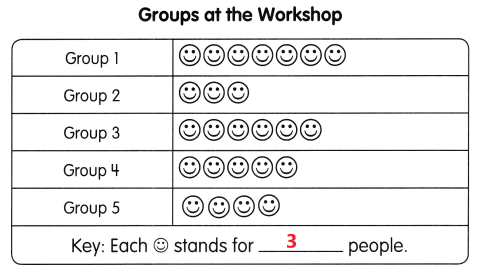Explanation:
Eachstands for 5 people
I need to represent 20 people in Group 5
So, i drew 44 x 5  20.

Question 35.
7 people in Group 3 were women. How many were men?
23 were men

Explanation:
There are 6in group 3
Eachstands for 5 people
6 x 5 = 30
7 people in Group 3 were women
30 – 7 = 23
So, 23 people in group 3 were men.

Question 36.
There were 50 men in Group 1 and Group 4. How many women were there in Group 1 and Group 4?
Eachstands for 5 people
There are 7in Group 1
There are 5in Group 4